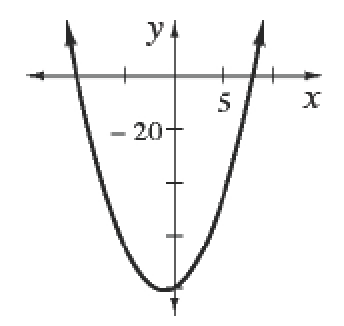### Home > INT3 > Chapter 3 > Lesson 3.1.4 > Problem3-66

3-66.

What are the $x$- and $y$-intercepts and the vertex of $y=x^2+2x-80$? Sketch the graph and write the equation in graphing form.

Set $x=0$ to determine the $y$-intercept.

Let $y=0$ and then factor and use the Zero Product Property to solve for the $x$-intercepts.

The vertex is $h$ and $k$ when the equation is in graphing form.

$y=(x+1)^2−81$